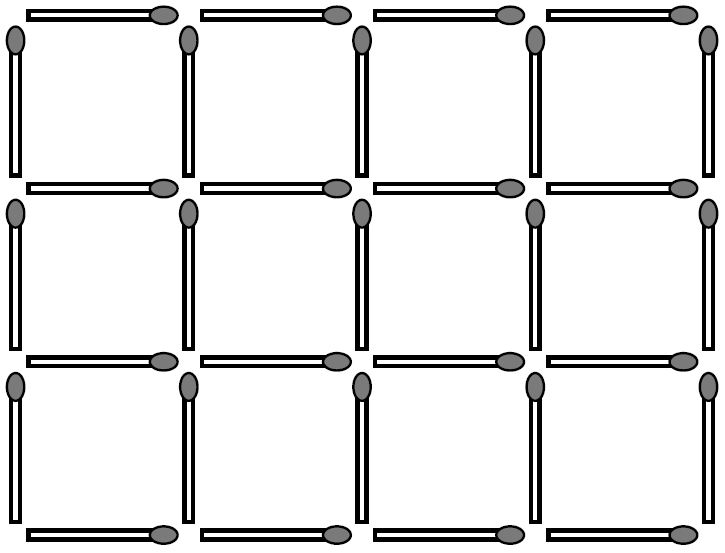## amc-2014-im-29

As shown in the diagram, you can create a grid of squares 3 units high and 4 units wide using 31 matches.I would like to make a grid of squares $$a$$ units high and $$b$$ units wide, where $$a<b %speech% ,a less than b,$$ are positive integers.

Determine the sum of the areas of all such rectangles that can be made, each using exactly 337 matches.